# Palindrome: Java program to check number is palindrome or not

How to find if number is palindrome in Java
Checking whether a number is palindrome or not is a classical Java homework exercise. When you start learning Java programming most institute which teach Java  programming provides these classical Java programs like How to find Armstrong number in Java or how to find Fibonacci number in Java using recursion etc. Write a Java program to check if number is palindrome comes from same category. For those who are not familiar with palindrome numbers, palindrome number is a number which is equal to reverse of itself. For example 121 is a palindrome because reverse of 121 is 121, while 123 is not a palindrome in Java because reverse of 123 is 321 and 121!=321. Finding palindrome number is easy in Java, you just need to develop logic to reverse a number in Java. Thankfully Java provides couple of arithmetic operators like remainder(%) operator also known as modules operator, which returns remainder in division operator and division operator(/) which returns quotient. By using remainder and division operator in Java we can create program to check if number is palindrome or not.

## Java program – palindrome numbers in JavaHere is a complete Java program to check if a given number is palindrome or not, This program works for both positive and negative numbers and display if its palindrome irrespective of there sign. Any one digit number including zero is always palindrome. This program is able to check two digit , three digit numbers for palindrome and effective go to any number in Java. Let’s see how to write Java program to find palindrome numbers :

package testing;
import java.util.Scanner;
/**
* Java program to check if number is palindrome or not.
* A number is called palindrome if number and its reverse is equal
* This Java program can also be used to reverse a number in Java
*/

public class NoClassDefFoundErrorDueToStaticInitFailure {

public static void main(String args[]){

System.out.println("Please Enter a number : ");
int palindrome = new Scanner(System.in).nextInt();

if(isPalindrome(palindrome)){
System.out.println("Number : " + palindrome + " is a palindrome");
}else{
System.out.println("Number : " + palindrome + " is not a palindrome");
}

}

/*
* Java method to check if number is palindrome or not
*/

public static boolean isPalindrome(int number) {
int palindrome = number; // copied number into variable
int reverse = 0;

while (palindrome != 0) {
int remainder = palindrome % 10;
reverse = reverse * 10 + remainder;
palindrome = palindrome / 10;
}

// if original and reverse of number is equal means
// number is palindrome in Java
if (number == reverse) {
return true;
}
return false;
}

}

Output:
Please Enter a number :
123
Number : 123 is not a palindrome
Please Enter a number :
121
Number : 123 is a palindrome

That’s all on how to check if a number is palindrome or not in Java. If you are looking answer of this question for interview purpose then better prepare a recursive version of palindrome program in Java as well because its common practice that programmer are expected to write java program using both recursion and iteration in Java. Let me know if you find any other way of checking palindrome in Java.

Further Learning
Data Structures and Algorithms: Deep Dive Using Java
Java Fundamentals: The Java Language
Complete Java Masterclass

Other Java homework programming exercise:

1.Very good Program!
Please correct the out put when it asks 121 is a palindrome....the result says
123" is a palindrome... it should be 121 :)
Cheers!

2.Hi, Thanks for this. The program is working well but I am trying to understand how the reverse(sort of where it came from) fits the program.

3.// Variations, using charAt

public static boolean isPolindrome(int n) {

String s = String.valueOf(n).toString();
String rs = new StringBuffer(s).reverse().toString();

for (int i=0; i<s.length(); i++) {
if (s.charAt(i) != rs.charAt(i)) return false;
}

return true;
}

4.Hi you dont even need to reverse the string .

static boolean checkAlgoOne(int num) {

boolean check = false;

String number = Integer.toString(num);
char[] chars = number.toCharArray();
int len = chars.length;

if (num < 0)
check = false;
if (num == 0 || num == 1)
check = true;

if (len % 2 != 1) {
check = false;
} else {

for (int i = 0; i <= len / 2; i++) {
if (chars[i] == chars[len - 1])
check = true;
}

}
return check;
}

cheers!

1.1221 is palindrome, but your program will say it's not

5.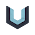My way:
/* Palindrome Program In JAVA
© Code Nirvana (www.codenirvana.in)
*/
import java.util.Scanner;
class Palindrome{
public static void main(String args[]){
System.out.print("Enter Number: ");
Scanner read = new Scanner(System.in);
int num = read.nextInt();
int n = num;
//reversing number
int rev=0,rmd;
while(num > 0)
{
rmd = num % 10;
rev = rev * 10 + rmd;
num = num / 10;
}
if(rev == n)
System.out.println(n+" is a Palindrome Number!");
else
System.out.println(n+" is not a Palindrome Number!");
}
}

MORE @ http://www.codenirvana.in/2013/10/Palindrome-Number-in-JAVA.html

1.hi,

can u explain why you have divided input by 10 and and %10 for reversing a number series...please explain that part.

2.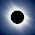Hello @Anonymous, when you divide the number you drop last digit from number and when you to module 10 i.. % 10 you get the last digit of the number.

For example, 123/10 = 12, last digit 3 is gone so number reduced from 3 to 2
123%10 = 3 means last digit.

This is the trick you need to reverse the number.

6.private static boolean isPalindrome(int n) {
return n == Integer.valueOf(new StringBuilder(String.valueOf(n)).reverse().toString());
}

7.private void checkPolyndrom(String st) {
boolean polyn=true;
for(int i =0; i<st.length()-1/2; i++){
if(st.charAt(i)==st.charAt(st.length()-1-i) && polyn){
polyn=true;
}else{
polyn=false;
}
}
if(polyn){
System.out.println("Polyndrom");
}else{
System.out.println("Not Polyndrom");
}

}

8.Why do we multiply rev*10+ remainder

1.9.all are from which standards

10.Is 1221 a palindrome number. ????

1.11.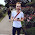public static boolean isPalindrome(int n) {
String i = Integer.toString(n);
int first = 0;
int last = i.length() - 1;

while (first < last) {
if (i.charAt(first) == i.charAt(last)) {
first++;
last--;
} else {
return false;
}
}
return true;
}

12.good examples and thanks to all..

13.how to close the scanner int function?? for resolving warning.

1.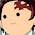(Scanner name).close();

2.myScanner.close();

14.try this simple way

import java.util.*;
class StringPalindrome
{
public static void main (String ar[])
{
String a, b;
StringBuffer sb;
Scanner sc=new Scanner (System.in);
System.out.println("Ente rhe string");
a=sc.nextLine();
sb=new StringBuffer(a);
b=sb.reverse().toString();
if(a.equals(b))
{
System.out.println("The string is palidrome");
}
else
{
System.out.println("The string is not a palindrome");
}}}

15.public static boolean isNumberPalindrome(int str) {

return str == Integer.parseInt(new StringBuilder().append(str).reverse().toString());
}

16.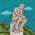Palindrome Check using HashSet:
import java.util.HashSet;
import java.util.Set;

public class p16 {

public static void main(String[] args) {
String a = "malayalam1";

System.out.println(ispalindrome(a));

}

private static boolean ispalindrome(String a) {

Set set = new HashSet<>();
for (int i = 0; i < a.length(); i++) {
char ch = a.charAt(i);
if(set.contains(ch)) {
set.remove(ch);
}else {
}
}
return set.size() <= 1 ;

}

}

17.Integer i = 1221;
char word[] = i.toString().toCharArray();

int i1 = 0;
int i2 = word.length - 1;
while (i2 > i1) {
if (word[i1] != word[i2]) {
System.out.println("not palindrome");
break;
}
++i1;
--i2;
}

18.if(i==s.length()%2)
{
System.out.println("Palidrome");
}